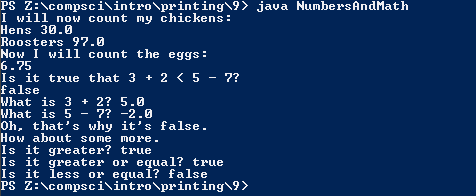# Assignemnt #11 and Numbers and Math

## Code

```    ///Name: Joshua Bautista
///Period: 6
///Project Name: Numbers and Math
///File Name: NumbersAndMath.java
///Date: 9/14/2015

public class NumbersAndMath
{
public static void main( String[] args )
{
System.out.println( "I will now count my chickens:" );
// Makes Hen into a sentence then computer does math inside the parenthesis
System.out.println( "Hens " + ( 25.0 + 30.0 / 6.0 ) );
//Makes roosters a sentence then does math inside the parenthesis
System.out.println( "Roosters " + ( 100.0 - 25.0 * 3.0 % 4.0 ) );

System.out.println( "Now I will count the eggs:" );
// Computer does math inside the parenthesis
System.out.println( 3.0 + 2.0 + 1.0 - 5.0 + 4.0 % 2.0 - 1.0 / 4.0 + 6.0 );
//Making a sentence in the stuff put around the quotes
System.out.println( "Is it true that 3 + 2 < 5 - 7?" );
//Asking computer to do the math inside parenthesis
System.out.println( 3.0 + 2.0 < 5.0 - 7.0 );
// Asking computer to do the math in the parenthesis makes stuff in quotes sentences
System.out.println( "What is 3 + 2? " + ( 3.0 + 2.0 ) );
//Asking computer to do the math in the parenthesis makes stuff in quotes sentences
System.out.println( "What is 5 - 7? " + ( 5.0 - 7.0 ) );

System.out.println( "Oh, that's why it's false." );

System.out.println( "How about some more." );
//Asking computer if 5>-2 which is true
System.out.println( "Is it greater? " + ( 5.0 > -2.0 ) );
//Asking computer if 5 >= -2 which is true
System.out.println( "Is it greater or equal? " + ( 5.0 >= -2.0 ) );
// Asking computer if 5 <=-2 which is false
System.out.println( "Is it less or equal? " + ( 5.0 <= -2.0 ) );
}
}

```

### Picture of the output